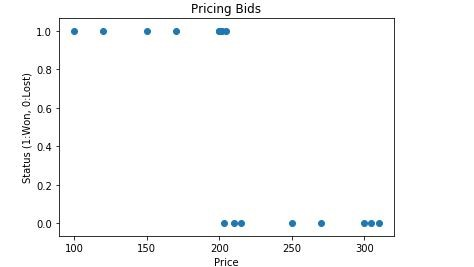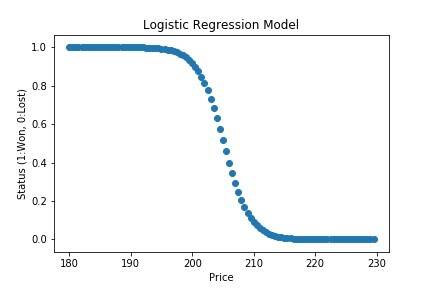Python Logistic Regression with SciKit Learn

Logistic regression is amongst the most commonly known “core” machine learning algorithms out there together with its cousin, Linear Regression. It has many applications in business one of which is in Pricing Optimization. In this article, you will learn how to code Logistic Regression in Python using the SciKit Learn library to solve a Bid Pricing problem.

What is Logistic Regression?

Logistic regression is a predictive linear model that aims to explain the relationship between a dependent binary variable and one or more independent variables. The output of Logistic Regression is a number between 0 and 1 which you can think about as being probability that a given class is true or not.

The reason the output is between 0 and 1 is because the output is transformed by a function which usually is the logistic sigmoid function.

The formula for Logistic Regression is the following:

• F(x)  = an ouput between 0 and 1
• x = input to the function
• m,b are learned parameters (slope and intercept)

In Logistic Regression, our goal is to learn parameters m and b, similar to Linear Regression. The difference being that for a given x, the resulting (mx + b) is then squashed by the sigmoid function returning a number between 0 and 1.

Generate Bid Pricing Data

Let’s generate a dataset that we will be using to learn how to apply Logistic Regression to a pricing problem. The bid price is contained in our X variable while the result, a binary Lost or Won category encoded as a 1 (won) or 0 (lost), is hold in our Y variable.

x = np.array([100,120,150,170,200,200,202,203,205,210,215,250,270,300,305,310])
y = np.array([1,1,1,1,1,1,1,0,1,0,0,0,0,0,0,0])

Let’s go ahead and plot this using MatplotLib to gain a better understanding of our dataset.

import matplotlib.pyplot as plt
import numpy as np

plt.scatter(x,y)
plt.title("Pricing Bids")
plt.xlabel('Price')
plt.ylabel('Status (1:Won, 0:Lost)')Each point above represents a bid that we participated in. On the X axis you see the price that was offered and on the Y axis you see the result, if we won the bid or lost it. Our goal is to use Logistic Regression to come up with a model that generates the probability of winning or losing a bid at a particular price.

Logistic Regression with Sklearn

In python, logistic regression is made absurdly simple thanks to the Sklearn modules. For the task at hand we will be using LogisticRegression module.

First step, import the required class and instantiate a new LogisticRegression class.

from sklearn.linear_model import LogisticRegression
logreg = LogisticRegression(C=1.0, solver='lbfgs', multi_class='ovr')

The LogisticRegression class requires some attributes. We keep the default inverse of regularization strength (C)  to 1.0. For the solver we use lbfgs. For multi-class we specify ovr as we are dealing with a binary classification problem. If you want to take a further look at the options available click on here.

The next step is to fit the logistic regression model by running the fit function of our class. Before we can do that though, we transform our x array into a 2D array as is required by the sklearn model. This is because we have only 1 feature (price). If we had more than 1 feature, our array would already be 2D.

Don’t skip this step otherwise you will see the following error: "ValueError: Expected 2D array, got 1D array instead"

#Convert a 1D array to a 2D array in numpy
X = x.reshape(-1,1)

Finally, fit your model.

#Run Logistic Regression
logreg.fit(X, y)

Make Predictions

There are 2 ways to generate predictions from your fit model. The first one I will show returns the predicted label. In our case, 1 for won and 0 for loss.

To predict the binary class, use the predict function like below.

logreg.predict(X)
#array([1, 1, 1, 0, 0, 0, 0, 0, 0, 0, 0, 0, 0, 0, 0, 0])

If we wanted to run the prediction on a specific price you can do so as below. Notice the double brackets next to ur price needed to convert into a 2d array.

print(logreg.predict([]))
print(logreg.predict([]))
#
#

Sklearn Predict Probabilities

The other method to make predictions using the logistic regression function is using the predict_proba function. This function instead of returning the predicted label, returns the models probability for the given input.

print(logreg.predict_proba([]))
print(logreg.predict_proba([]))
#[[0.08194444 0.91805556]]
#[[0.90780381 0.09219619]]

As you can tell above, the predict_proba function returns 2 values corresponding to the probability of the 0 label and the 1 label correspondingly. Reading above, we have that at the price of $200 we have an 8% probability of losing the bid (label 0) and a 91.8% probability of winning the bid (label 1). At$210, there is a bid shift with just a 10 difference in the price. Our model predicts a 90.7% probability of losing the bid and a 9% probability of losing the bid.

Thanks to the power of Logistic Regression, if you encountered this problem in real life you can use this model to help you optimize the pricing.

Let’s now visualize our predictions in a chart.

Logistic Regression Pricing Model

The way we will graph our model is by generating a list of possible prices between 180 and 230. We will then loop through each price and generate the probability of winning at that price. We then take these 2 variables prices and probabilities and show them using a scatter plot in Matplotlib.

prices = np.arange(180, 230, 0.5)
probabilities= []
for i in prices:
p_loss, p_win = logreg.predict_proba([[i]])
probabilities.append(p_win)

With the prices and probabilities lists populated, let's see the scatter plot.

plt.scatter(prices,probabilities)
plt.title("Logistic Regression Model")
plt.xlabel('Price')
plt.ylabel('Status (1:Won, 0:Lost)')Conclusion

You have now learned how to use logistic regression in python using Scikit learn. We applied it to a bid pricing business problem in which we wanted to find the probability of making a sale at a specific price point. Our problem was a binary classification with one input feature, price. Lastly we generated a graph of our probabilities using matplotlib. I hope this simple example of logistic regression has been useful. Stay tuned for more!